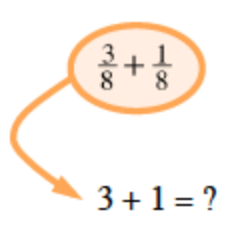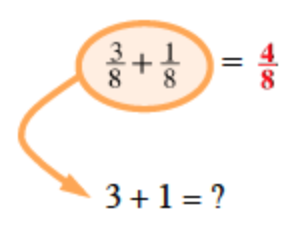### Home > MC2 > Chapter 1 > Lesson 1.2.3 > Problem1-79

1-79.

Rewrite each expression as a single fraction.

1. $\frac{3}{8}+\frac{1}{8}$

When adding or subtracting fractions with a common denominator, add or subtract with the numerators only. Circle around the problem with arrow pointing to 3 plus 1 = question mark.The problem now reads, 3 eighths + 1 eighth = 4 eighths. Reduce if possible.

1. $\frac{4}{5}+\frac{3}{4}$

See part (a).

1. $\frac{6}{7}-\frac{2}{5}$

See part (a).